## Straight-Line Depreciation

The most common method of depreciation used on a company's financial statements is the straight-line method. When the straight-line method is used each full year's depreciation expense will be the same amount.

We will illustrate the details of depreciation, and specifically the straight-line depreciation method, with the following example.

### Example of Straight-Line Depreciation

A company has decided that it wants to use the straight-line method for reporting depreciation on its financial statements. The company purchased equipment for use in its business operation and provides the following information:

• On July 1, 2021, the company purchased equipment for \$10,500

• The account Equipment was debited for \$10,500 and the account Cash was credited for \$10,500

• The company estimated that the equipment's salvage value at the end of its useful life will be \$500

• The company estimated that the equipment's useful life will be 5 years

Given the above information, the straight-line depreciation expense for each full year that the asset is used will be \$2,000 as calculated here: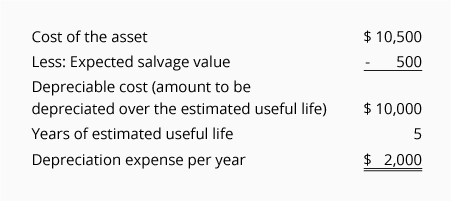If a company's accounting year ends on December 31, the company's income statement will report the depreciation expense as follows: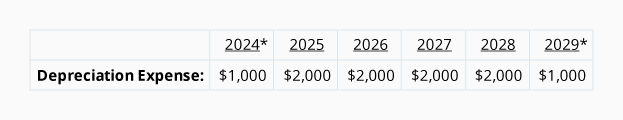*Since the asset was acquired on July 1, 2021, only half of the annual depreciation expense amount is recorded in 2021 and 2026.

The company's cash payment for the equipment took place on a single day in 2021 as shown here:Since depreciation expense is reported in all years from 2021 through 2026, but the cash payment took place only at the time when the equipment was purchased, each year's depreciation expense is often described as a noncash expense.

### Recording Straight-Line Depreciation

Depreciation is recorded in the company's accounting records through adjusting entries. Adjusting entries are recorded in the general journal using the last day of the accounting period.

Assuming the company prepares only annual financial statements for its years that end on December 31, the adjusting entries will be as follows:If a company issues monthly financial statements, the amount of each monthly adjusting entry will be \$166.67.

### Visualizing the Balances in Equipment and Accumulated Depreciation

Note that the account credited in the above adjusting entries is not the asset account Equipment. Instead, the credit is entered in the contra asset account Accumulated Depreciation. The use of this contra account allows the asset account Equipment to continue to report the equipment's cost, while also reporting in Accumulated Depreciation the total amount of depreciation expense that has been reported since the asset was acquired.

To assist in visualizing the balances in the asset account Equipment and the related contra asset account Accumulated Depreciation as of December 31, 2022 we are providing the following T-accounts:### Book Value or Carrying Value of Assets

The combination of an asset account's debit balance and its related contra asset account's credit balance is the asset's book value or carrying value.

Using the account balances in the T-accounts above, the book value or carrying value of the company's equipment as of December 31, 2022 is: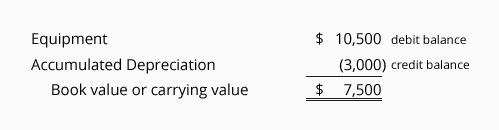When the asset's book value is equal to the asset's estimated salvage value, the depreciation entries will stop. If the asset continues in use, there will be \$0 depreciation expense in each of the subsequent years. The asset's cost and its accumulated depreciation balance will remain in the general ledger accounts until the asset is disposed of.

### Depreciation is Based on Estimates

It is important to realize that the amount of depreciation reported by a company is an estimated amount. The reason is that the calculation of depreciation uses the following estimates:

• Salvage value
An asset's salvage value is also described as the asset's disposal value, scrap value or residual value. Salvage value is an estimate of the amount the company expects to receive when it disposes of the asset at the end of the asset's useful life. (It is common for companies to assume that an asset will have no salvage value.)

• Useful life
The useful life of an asset is an estimate of how long the asset is expected to be used in the business. For example, a design engineer might purchase a new computer and estimate that the computer will be useful in the business for only 2 years (due to rapid advances in software and hardware). At the same time, an accountant might purchase a similar computer and estimate that it will be useful in the accounting business for 4 years. Both the design engineer's estimated useful life of 2 years and the accountant's estimated useful life of 4 years are correct (even though the computers are similar and may have a physical life of more than 10 years).

### What Happens When an Estimated Amount Changes

For financial statements to be relevant for their users, the financial statements must be distributed soon after the accounting period ends. To achieve this requirement, accountants must estimate some amounts.

After the financial statements are distributed, it is reasonable to learn that some actual amounts are different from the estimated amounts that were included in the financial statements. Unless the differences are significant no action is required.

If there is a significant change in an asset's estimated salvage value and/or the asset's estimated useful life, the change in the estimate will result in a new amount of depreciation expense in the current accounting year and in the remaining years of the asset's useful life.

NOTE:
A change in the estimated salvage value or a change in the estimated useful life of an asset that is being depreciated is not considered to be an accounting error. As a result, the financial statements that have already been distributed are not changed.

A significant change in the estimated salvage value or estimated useful life will be reported in the current and remaining accounting years of the asset's useful life.

### Example of a Change in the Estimated Useful Life of an Asset

To illustrate a change in the estimated useful life of an asset, we will assume a company had the following situation:

• Equipment was purchased on January 1, 2017 at a cost of \$14,000

• The company originally estimated the equipment will have no salvage value

• The company originally estimated that the equipment's useful life was 7 years

• Straight-line depreciation was used (resulting in depreciation of \$2,000 in each full year)

• In 2021 the company realized that the equipment would not be useful after December 31, 2022 (instead of December 31, 2023)

• The estimated salvage value at the end of the equipment's useful life remains at \$0

• Instead of the original useful life of 7 years (January 1, 2017 through December 31, 2023), the company now estimates a total useful life of only 6 years (January 1, 2017 through December 31, 2022)

• The depreciation already reported for the years 2017 through 2020 cannot be changed since the change is not an accounting error

• The change in the estimated useful life will affect only the depreciation being reported for 2021 and 2022

Let's first review the original straight-line depreciation using the estimates in January 2017: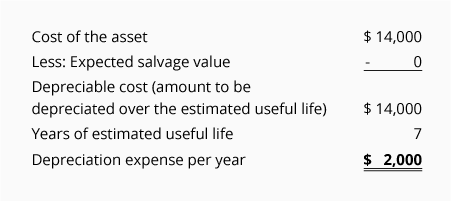Note in the following T-accounts that on December 31, 2020, the balance in the Equipment account is \$14,000 (the cost of the equipment) and the account Accumulated Depreciation has a credit balance of \$8,000: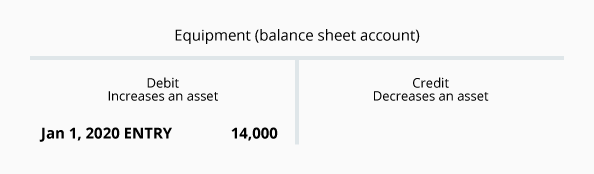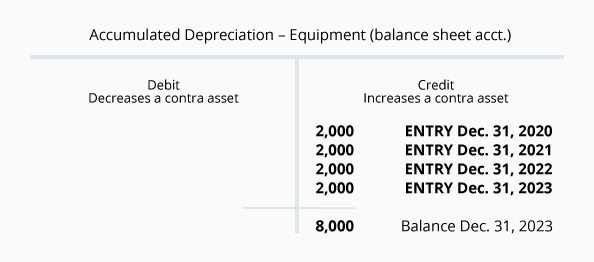The above accounts indicate that the book value of the equipment as of December 31, 2020 is \$6,000 (\$14,000 – \$8,000). We also know that only two years remain (2021 and 2022) in which to depreciate the remaining \$6,000 of book value. Since, the estimated salvage value is \$0, the remaining \$6,000 is divided by the 2 years remaining = \$3,000 of depreciation expense in each of the years 2021 and 2022.

The adjusting entries for 2021 and 2022 are as follows: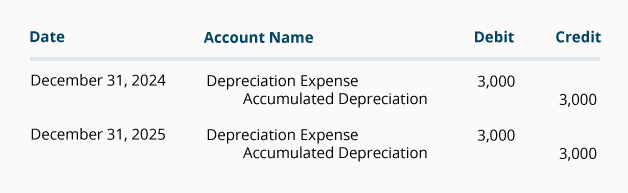As of December 31, 2022, the Accumulated Depreciation account will look like this: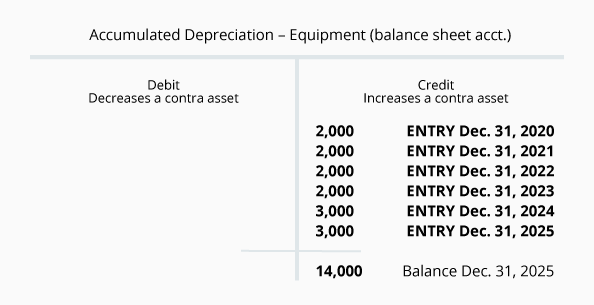Note that the depreciation amounts recorded in the years 2020 and before were not changed.

Now that you have learned the basic concepts of the depreciation reported on a company's financial statement, we will move on to calculate depreciation using three additional depreciation methods:

• Units-of-activity (or units of production)
• Double-declining-balance
• Sum-of-the-years'-digits